## 1 Introduction

This article provides information on the equation describing conservation of energy relevant to ﬂuid dynamics and computational ﬂuid dynamics (CFD). It ﬁrst assembles an equation for combined mechanical and thermal energy, i.e. total energy, in terms of material derivatives. It then presents an equation for thermal, or internal, energy. The total energy equation is then provided in terms of local (partial) derivatives, both in terms of internal energy and enthalpy. The implementation of the energy equation in solvers in OpenFOAM is then described.

## 2 Total Energy

The law of conservation of energy states that the total energy of an isolated system remains constant, i.e. it is conserved over time and energy is not created or destroyed but is transformed from one form to another. Here we consider only mechanical and thermodynamic energy, the contributions of which are described in the following sections, using usual notation of tensor algebra and calculus, including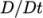representing the material derivative.

### 2.1 Mechanical Power

The rate of change of mechanical, or kinetic, energy is: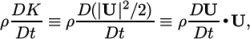(1)
where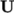is velocity, speciﬁc kinetic energy (kinetic energy per unit mass)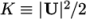and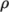is mass density. The power ﬂux, or rate of change of strain energy, is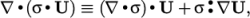(2)
where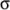is the mechanical stress tensor. The stress tensor may be decomposedinto a scalar thermodynamic pressureand viscous stress tensorby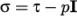, whereis the identity tensor. The power source, or rate of change of potential energy, is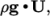(3)
where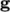is a body acceleration, e.g. gravity.

### 2.2 Thermodynamic Power

The rate of change of thermal, or internal, energy is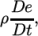(4)
where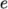is speciﬁc internal energy (internal energy per unit mass). The heat ﬂux is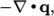(5)
where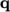is the heat ﬂux vector, deﬁned positive inwards. The heat source is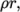(6)
where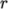is any speciﬁc heat source.

### 2.3 Conservation of Energy

The rate of change of total energy for a particle of material must equal the input of mechanical and thermodynamic power from ﬂuxes and sources acting on the particle. In the limit where particle size is inﬁnitesimally small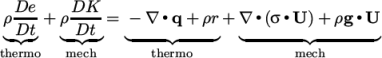(7)

## 3 Internal Energy

An equation for internal energy is produced by simplifying the mechanical contributions which, expressed as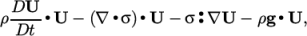(8)
combines with the momentum equation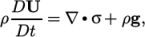(9)
to reduce the mechanical contributions to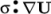. Equation 7 can then be expressed as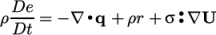(10)
The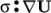term represents the contribution of mechanical power to internal energy, and thus, random motion of particles. The expression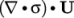must then represent a power due bulk motion of particles.

## 4 Total Energy/Enthalpy, local derivatives

We can express our equations in terms of the local derivative (or partial derivative, spatial derivative, …)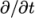, where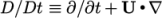. Applying conservation of mass, the following relationship holds for any tensor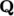: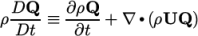(11)

Combining equations 7 and 11, and decomposing the stress tensor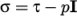, gives: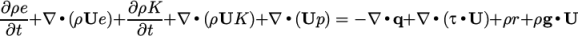(12)

Enthalpy is the sum of internal energy and kinematic pressure, i.e.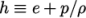. Combining this with equation 12 gives: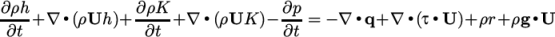(13)

Total energy can be deﬁned as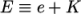. Combining this with equation 12 gives: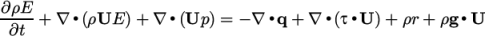(14)

## 5 Energy Equation in OpenFOAM Solvers

The solution of the energy equation is included in several solvers in OpenFOAM for compressible ﬂow, combustion, heat transfer, multiphase ﬂow and particle tracking. The source code can be found for these solvers within ﬁles in sub-directories of the \$FOAM_SOLVERS directory of OpenFOAM (including the compressible, combustion, heatTransfer, multiphase and lagrangian sub-directories).

The energy equation is generally implemented in the form of total energy expressed in equations 12 and 13, without the mechanical sources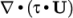and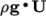. A heat ﬂux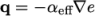is assumed, where the eﬀective thermal diﬀusivity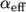is the sum of laminar and turbulent thermal diﬀusivities. The implementation of each energy equation contains thermal source terms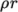relevant to the particular solver.

For example, the sonicFoam solver contains the following implementation of the energy equation from equation 12.

fvScalarMatrix EEqn
(
fvm::ddt(rho, e) + fvm::div(phi, e)
+ fvc::ddt(rho, K) + fvc::div(phi, K)
+ fvc::div(fvc::absolute(phi/fvc::interpolate(rho), U), p, "div(phiv,p)")
- fvm::laplacian(turbulence->alphaEff(), e)
==
fvOptions(rho, e)
);

sonicFoam solves equations sequentially, so solves the momentum equation for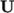before updating the speciﬁc kinetic energy ﬁeld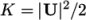for the energy equation above. More commonly, the energy equation is implemented in terms of both internal energy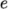and enthalpy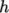, as both equations 12 and 13, allowing the user to choose the solution variable,or, at run time. For example, the rhoPimpleFoam solver has the following implementation:

volScalarField& he = thermo.he();

fvScalarMatrix EEqn
(
fvm::ddt(rho, he) + fvm::div(phi, he)
+ fvc::ddt(rho, K) + fvc::div(phi, K)
+ (
he.name() == "e"
? fvc::div
(
fvc::absolute(phi/fvc::interpolate(rho), U),
p,
"div(phiv,p)"
)
: -dpdt
)
- fvm::laplacian(turbulence->alphaEff(), he)
==
fvOptions(rho, he)
);

Here, “he” represents either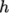or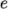. The 5th term switches between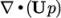and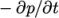depending on the solution variable chosen by the user.

The rhoCentralFoam solver includes an implementation of an energy equation best represented by equation 14 that includes the mechanical source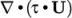.

## 6 Total vs Internal Energy

The choice of energy equation has a signiﬁcant on some solutions particularly across shocks. In the well known 1D shockTube tutorial example (Sod’s problem), the initial discontinuity causes a shock to propagate into the low pressure region and an expansion wave to propagate upstream. The ﬁgure below shows the temperature after 0.007 s, with simulation results compared with the analytical solution. Using the version of sonicFoam prior to OpenFOAM v2.2.0 that solves a thermal energy equation, the temperature diﬀerence across the shock is badly predicted. Using sonicFoam from v2.2.0 onwards that solves a total energy equation, conservation of total energy ensures the temperature diﬀerence is predicted accurately.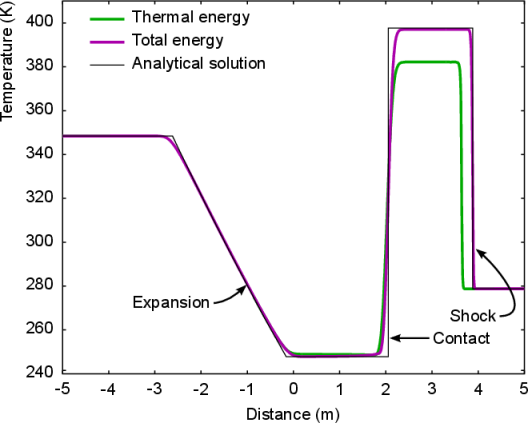Figure 1: Shock tube problem, solution at 0.007s

Energy Equation in OpenFOAM